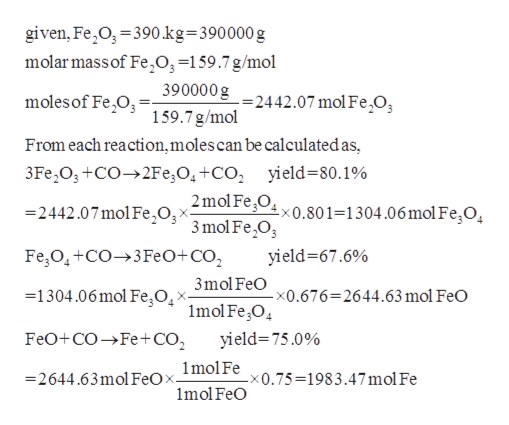# A three-step process for producing molten iron metal from Fe2O3 is:3Fe2O3 + CO → 2Fe3O4 + CO2Fe3O4 + CO → 3FeO + CO2FeO + CO → Fe + CO2Assuming that the reactant CO is present in excess and that the yields, respectively, for the three steps are 80.1%, 67.6% and 75.0%, what mass of iron metal would be produced from 390. kg of Fe2O3?

Question
12 views

A three-step process for producing molten iron metal from Fe2O3 is:

1. 3Fe2O3 + CO → 2Fe3O4 + CO2
2. Fe3O4 + CO → 3FeO + CO2
3. FeO + CO → Fe + CO2

Assuming that the reactant CO is present in excess and that the yields, respectively, for the three steps are 80.1%, 67.6% and 75.0%, what mass of iron metal would be produced from 390. kg of Fe2O3?

check_circle

Step 1

The moles of iron produced from 390.kg o...help_outlineImage Transcriptionclosegiven, Fe O, 390.kg=390000 g molar massof Fe,03=159.7 g/mol 390000g-2442.07 mol Fe,O, 159.7 g/mol moles of Fe O From each reaction,moles can be calculatedas, 3Fe2O+CO2Fe,O4+CO2 yield=80.1% 2mol FeO, x0.80 1=1304.06 mol FegO, 2442.07mol FeO;^ 3mol Fe,03 Fe O4+CO3FeO+CO2 yield-67.6% 3mol FeO =1304.06 mol Fe O -x0.676=2644.63 mol FeO X 1mol Fe,O yield 75.0% FeO+COFe+CO2 2644.63mol FeOx_lmolFex0.75=1983.47 mol Fe 1mol FeO fullscreen

### Want to see the full answer?

See Solution

#### Want to see this answer and more?

Solutions are written by subject experts who are available 24/7. Questions are typically answered within 1 hour.*

See Solution
*Response times may vary by subject and question.
Tagged in

### Physical Chemistry